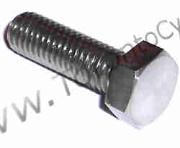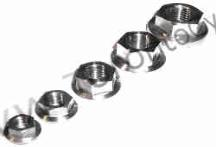Titanium US Nuts
 Pitch:1/4" = 20tpi5/16 = 18tpi3/8" = 16tpi
 1/4" Nut 2.7 5/16 Nut 3.2 3/8" Nut 3.6Titanium Washers
 1/4" = 1.25 5/16"= 1.4 3/8" = 1.6Unified National Fine (UNF)Titanium US Nuts
 1/4" Nut 2.7 5/16 Nut 3.2 3/8  Nut 4 1/2" Nut 4.8Fine Pitch:1/4" = 28tpi5/16 = 24tpi3/8" = 24tpiUnified National Coarse (UNC)Ti Hex Head US Titanium Bolts1/4"x 0.5" = 3.3 1/4"x 0.75" = 3.6 1/4"x 1" = 3.9 1/4"x 1.5" =Threaded Section 1" 4.3
 Pitch:1/4" = 20tpi5/16 = 18tpi3/8" = 16tpiUnified National Coarse (UNC)Ti Hex Head US Titanium Bolts5/16"x 0.5" = 3.8 5/16"x 0.75" = 4.3 5/16"x 1" = 4.3 5/16"x 1.5" =Threaded Section 1" 4.8
 Pitch:1/4" = 20tpi5/16 = 18tpi3/8" = 16tpiUnified National Coarse (UNC)Ti Hex Head US Titanium Bolts3/8"x 0.5" = 5.2 3/8"x 0.75" = 5.5 3/8"x 1" = 5.8 3/8"x 1.5" =Threaded Section 1" 6
 Pitch:1/4" = 20tpi5/16 = 18tpi3/8" = 16tpi1/4"x 0.5" = 3.5 1/4"x 1" = 3.9
 UNC Pitch:1/4" = 20tpi5/16 = 18tpi3/8" = 16tpi
 5/16"x 0.5" = 3.8 5/16"x 1" = 4.2 5/16"x 1.5" = 4.5 3/8"x 1" = 6
 3/8"x 1.5" = 6.5 3/8"x 2" = 7Unified National Coarse (UNC)Ti Non-Taper Head Titanium US Bolts
 Unified National Fine (UNF)Ti Non-Taper Head Titanium US Bolts
 UNF Pitch:1/4" = 28tpi5/16 = 24tpi3/8" = 24tpi
 3/8"x 1.0" = 5.9 3/8"x 1.5" = 6.5 3/8"x 2" = 71/4"x 0.5" = 3.3 1/4"x 0.75" = 3.6 1/4"x 1.0" = 45/16"x 0.5" = 3.8 5/16"x 0.75" = 4 5/16"x 1.0" = 4.3Unified National Fine (UNF)Titanium US Lock Nuts
 Fine Pitch:1/4" = 28tpi5/16 = 24tpi3/8" = 24tpi
 5/16" Lock Nut 3.25Titanium US Flange Nuts
 3/8" Flange Nut26 Threads Per Inch 4.25Shipping InformationPlease check out FAQ page or Blog for shipping rates and information.
 Please Note that Tracking From Canada is Costly and Extra Charges Apply
 All Prices are in US Dollar\$5.00 Shipping via Air MailTracking is extra Costs!
 Some browsers like FireFox and Opera may not operate properly with checkout.If the cart button is missing then I am out of that item
 Please refresh browsers (F5 Key) as I update this website dailyUnified National Coarse (UNC)Ti Taper Head US Titanium Bolts
 1/4"x 1" = 3.8 1/4"x 1.5" = 4.35/16"x 0.5"= 3.8 5/16"x 3/4"= 4 5/16"x 1"= 4.4 5/16"x 1.5"= 4.8
 3/8"x 1"= 6 3/8"x 1.5"= 6.5
 Pitch:1/4" = 20tpi5/16 = 18tpi3/8" = 16tpiToronto Bike Parts, Titanium Bolts,  Bicycle Parts and Accessories